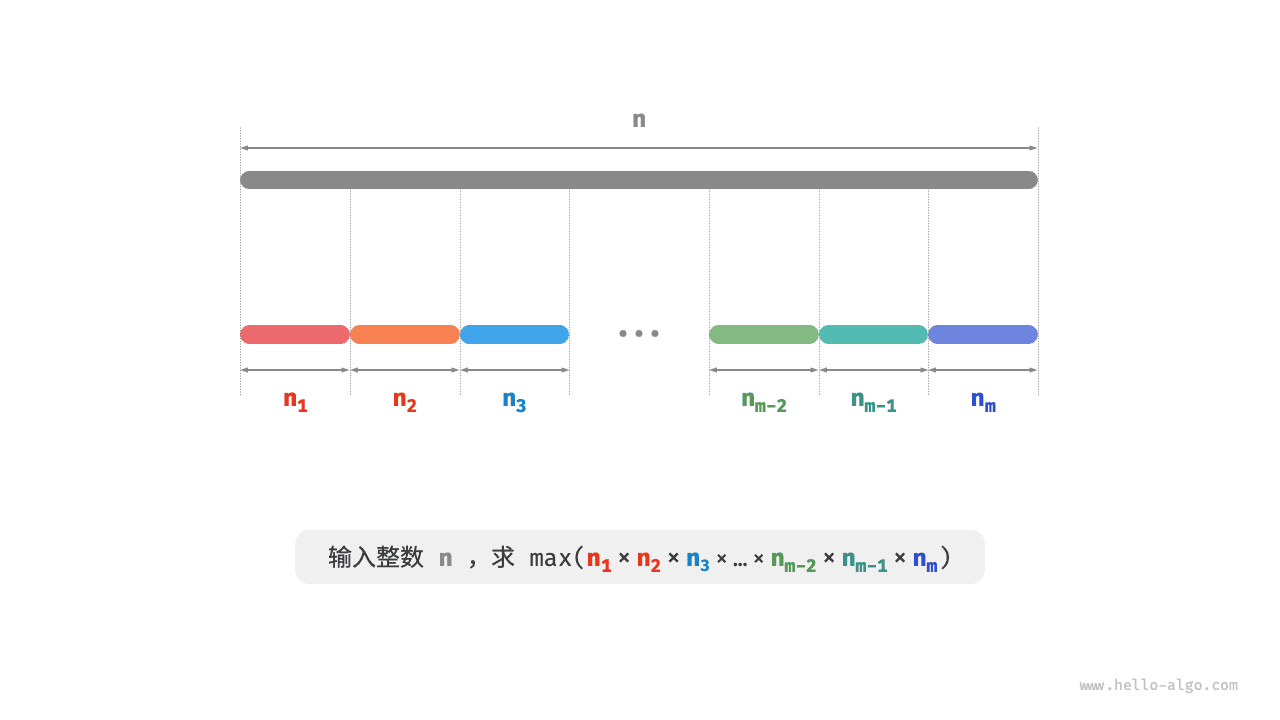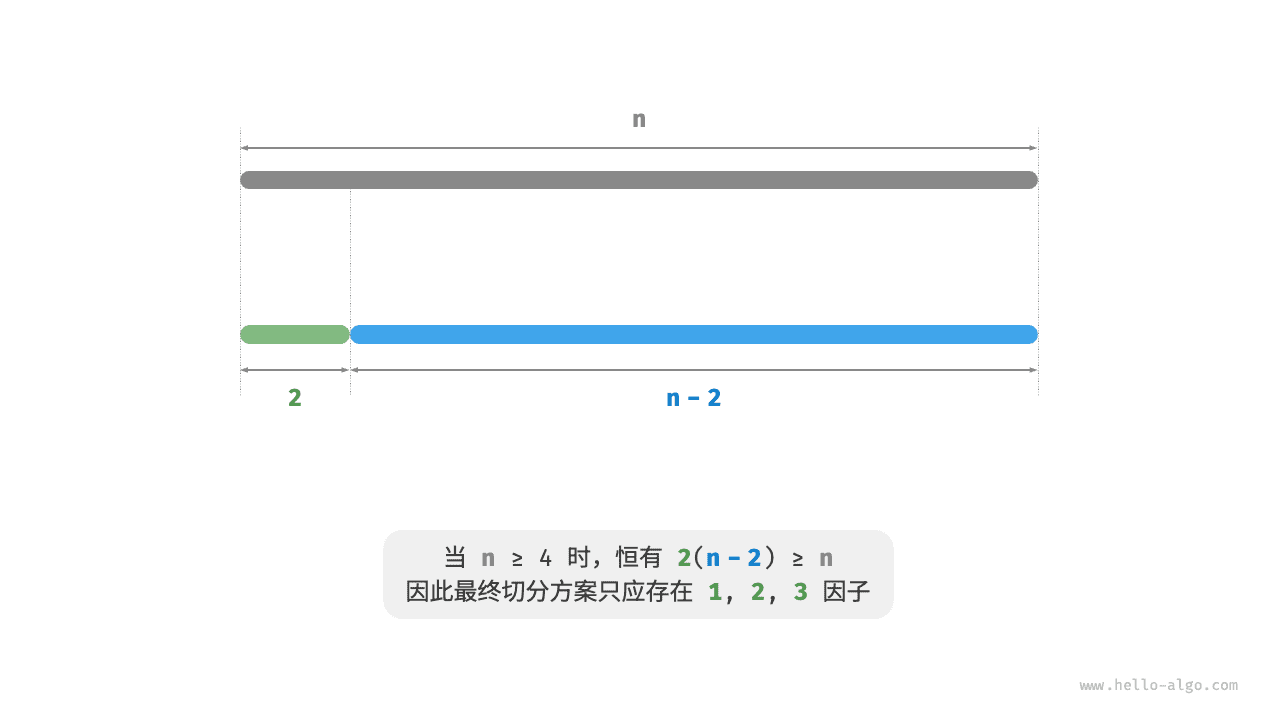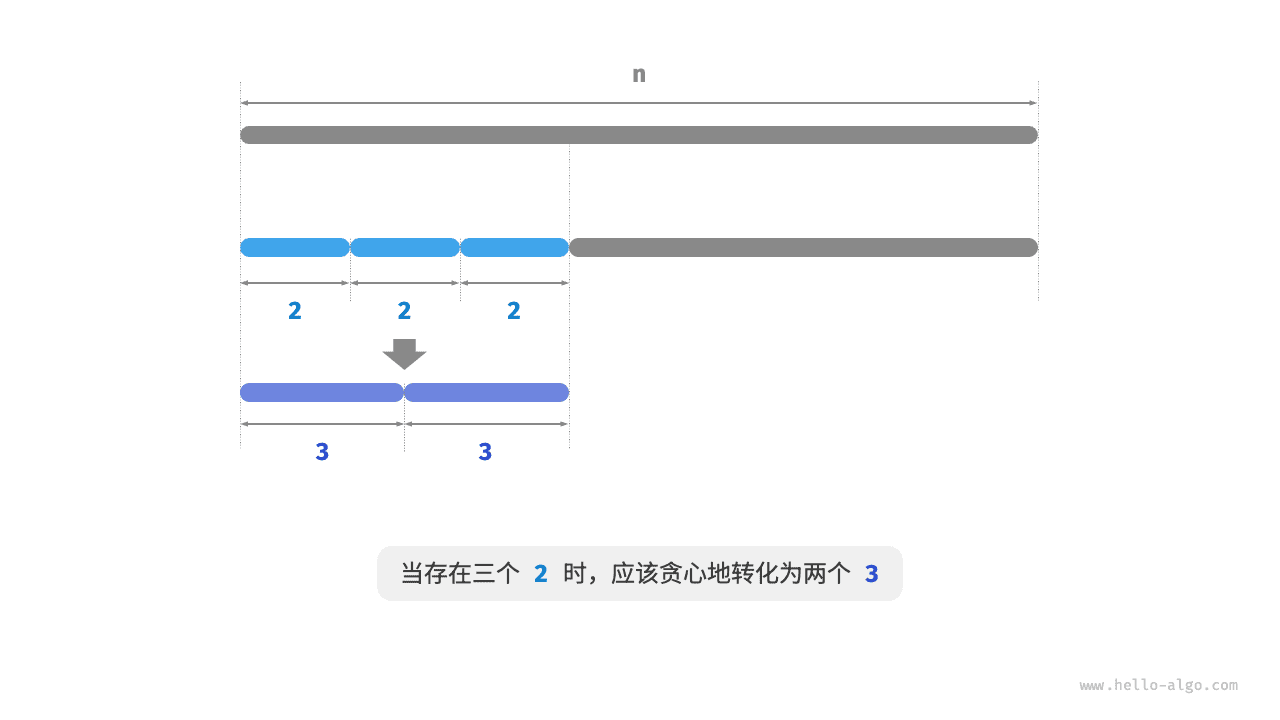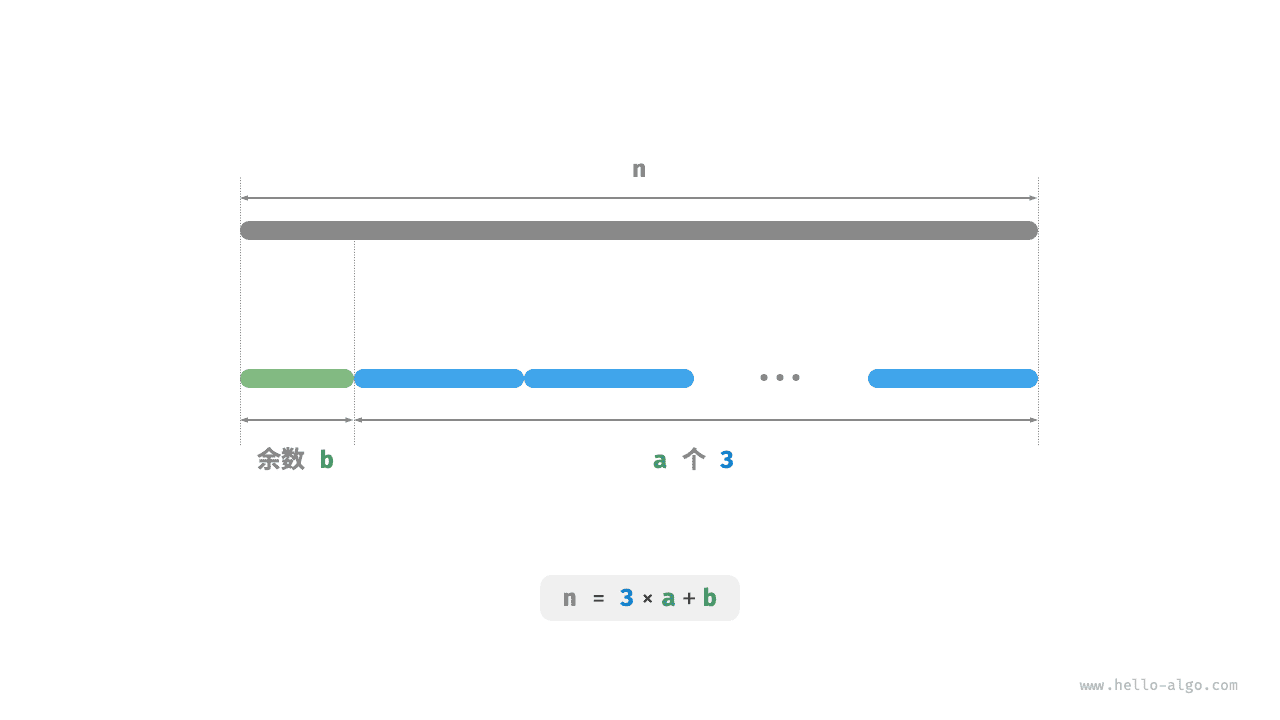# 15.4   最大切分乘积问题¶

Question$n = \sum_{i=1}^{m}n_i$

$\max(\prod_{i=1}^{m}n_i)$

### 1.   贪心策略确定¶

\begin{aligned} 2(n-2) & \geq n \newline 2n - n - 4 & \geq 0 \newline n & \geq 4 \end{aligned}1. 输入整数 $$n$$ ，从其不断地切分出因子 $$3$$ ，直至余数为 $$0$$$$1$$$$2$$
2. 当余数为 $$0$$ 时，代表 $$n$$$$3$$ 的倍数，因此不做任何处理。
3. 当余数为 $$2$$ 时，不继续划分，保留之。
4. 当余数为 $$1$$ 时，由于 $$2 \times 2 > 1 \times 3$$ ，因此应将最后一个 $$3$$ 替换为 $$2$$

### 2.   代码实现¶

$n = 3 a + b$

max_product_cutting.py
def max_product_cutting(n: int) -> int:
"""最大切分乘积：贪心"""
# 当 n <= 3 时，必须切分出一个 1
if n <= 3:
return 1 * (n - 1)
# 贪心地切分出 3 ，a 为 3 的个数，b 为余数
a, b = n // 3, n % 3
if b == 1:
# 当余数为 1 时，将一对 1 * 3 转化为 2 * 2
return int(math.pow(3, a - 1)) * 2 * 2
if b == 2:
# 当余数为 2 时，不做处理
return int(math.pow(3, a)) * 2
# 当余数为 0 时，不做处理
return int(math.pow(3, a))

max_product_cutting.cpp
/* 最大切分乘积：贪心 */
int maxProductCutting(int n) {
// 当 n <= 3 时，必须切分出一个 1
if (n <= 3) {
return 1 * (n - 1);
}
// 贪心地切分出 3 ，a 为 3 的个数，b 为余数
int a = n / 3;
int b = n % 3;
if (b == 1) {
// 当余数为 1 时，将一对 1 * 3 转化为 2 * 2
return (int)pow(3, a - 1) * 2 * 2;
}
if (b == 2) {
// 当余数为 2 时，不做处理
return (int)pow(3, a) * 2;
}
// 当余数为 0 时，不做处理
return (int)pow(3, a);
}

max_product_cutting.java
/* 最大切分乘积：贪心 */
int maxProductCutting(int n) {
// 当 n <= 3 时，必须切分出一个 1
if (n <= 3) {
return 1 * (n - 1);
}
// 贪心地切分出 3 ，a 为 3 的个数，b 为余数
int a = n / 3;
int b = n % 3;
if (b == 1) {
// 当余数为 1 时，将一对 1 * 3 转化为 2 * 2
return (int) Math.pow(3, a - 1) * 2 * 2;
}
if (b == 2) {
// 当余数为 2 时，不做处理
return (int) Math.pow(3, a) * 2;
}
// 当余数为 0 时，不做处理
return (int) Math.pow(3, a);
}

max_product_cutting.cs
/* 最大切分乘积：贪心 */
int MaxProductCutting(int n) {
// 当 n <= 3 时，必须切分出一个 1
if (n <= 3) {
return 1 * (n - 1);
}
// 贪心地切分出 3 ，a 为 3 的个数，b 为余数
int a = n / 3;
int b = n % 3;
if (b == 1) {
// 当余数为 1 时，将一对 1 * 3 转化为 2 * 2
return (int)Math.Pow(3, a - 1) * 2 * 2;
}
if (b == 2) {
// 当余数为 2 时，不做处理
return (int)Math.Pow(3, a) * 2;
}
// 当余数为 0 时，不做处理
return (int)Math.Pow(3, a);
}

max_product_cutting.go
/* 最大切分乘积：贪心 */
func maxProductCutting(n int) int {
// 当 n <= 3 时，必须切分出一个 1
if n <= 3 {
return 1 * (n - 1)
}
// 贪心地切分出 3 ，a 为 3 的个数，b 为余数
a := n / 3
b := n % 3
if b == 1 {
// 当余数为 1 时，将一对 1 * 3 转化为 2 * 2
return int(math.Pow(3, float64(a-1))) * 2 * 2
}
if b == 2 {
// 当余数为 2 时，不做处理
return int(math.Pow(3, float64(a))) * 2
}
// 当余数为 0 时，不做处理
return int(math.Pow(3, float64(a)))
}

max_product_cutting.swift
/* 最大切分乘积：贪心 */
func maxProductCutting(n: Int) -> Int {
// 当 n <= 3 时，必须切分出一个 1
if n <= 3 {
return 1 * (n - 1)
}
// 贪心地切分出 3 ，a 为 3 的个数，b 为余数
let a = n / 3
let b = n % 3
if b == 1 {
// 当余数为 1 时，将一对 1 * 3 转化为 2 * 2
return pow(3, a - 1) * 2 * 2
}
if b == 2 {
// 当余数为 2 时，不做处理
return pow(3, a) * 2
}
// 当余数为 0 时，不做处理
return pow(3, a)
}

max_product_cutting.js
/* 最大切分乘积：贪心 */
function maxProductCutting(n) {
// 当 n <= 3 时，必须切分出一个 1
if (n <= 3) {
return 1 * (n - 1);
}
// 贪心地切分出 3 ，a 为 3 的个数，b 为余数
let a = Math.floor(n / 3);
let b = n % 3;
if (b === 1) {
// 当余数为 1 时，将一对 1 * 3 转化为 2 * 2
return Math.pow(3, a - 1) * 2 * 2;
}
if (b === 2) {
// 当余数为 2 时，不做处理
return Math.pow(3, a) * 2;
}
// 当余数为 0 时，不做处理
return Math.pow(3, a);
}

max_product_cutting.ts
/* 最大切分乘积：贪心 */
function maxProductCutting(n: number): number {
// 当 n <= 3 时，必须切分出一个 1
if (n <= 3) {
return 1 * (n - 1);
}
// 贪心地切分出 3 ，a 为 3 的个数，b 为余数
let a: number = Math.floor(n / 3);
let b: number = n % 3;
if (b === 1) {
// 当余数为 1 时，将一对 1 * 3 转化为 2 * 2
return Math.pow(3, a - 1) * 2 * 2;
}
if (b === 2) {
// 当余数为 2 时，不做处理
return Math.pow(3, a) * 2;
}
// 当余数为 0 时，不做处理
return Math.pow(3, a);
}

max_product_cutting.dart
/* 最大切分乘积：贪心 */
int maxProductCutting(int n) {
// 当 n <= 3 时，必须切分出一个 1
if (n <= 3) {
return 1 * (n - 1);
}
// 贪心地切分出 3 ，a 为 3 的个数，b 为余数
int a = n ~/ 3;
int b = n % 3;
if (b == 1) {
// 当余数为 1 时，将一对 1 * 3 转化为 2 * 2
return (pow(3, a - 1) * 2 * 2).toInt();
}
if (b == 2) {
// 当余数为 2 时，不做处理
return (pow(3, a) * 2).toInt();
}
// 当余数为 0 时，不做处理
return pow(3, a).toInt();
}

max_product_cutting.rs
/* 最大切分乘积：贪心 */
fn max_product_cutting(n: i32) -> i32 {
// 当 n <= 3 时，必须切分出一个 1
if n <= 3 {
return 1 * (n - 1);
}
// 贪心地切分出 3 ，a 为 3 的个数，b 为余数
let a = n / 3;
let b = n % 3;
if b == 1 {
// 当余数为 1 时，将一对 1 * 3 转化为 2 * 2
3_i32.pow(a as u32 - 1) * 2 * 2
} else if b == 2 {
// 当余数为 2 时，不做处理
3_i32.pow(a as u32) * 2
} else {
// 当余数为 0 时，不做处理
3_i32.pow(a as u32)
}
}

max_product_cutting.c
/* 最大切分乘积：贪心 */
int maxProductCutting(int n) {
// 当 n <= 3 时，必须切分出一个 1
if (n <= 3) {
return 1 * (n - 1);
}
// 贪心地切分出 3 ，a 为 3 的个数，b 为余数
int a = n / 3;
int b = n % 3;
if (b == 1) {
// 当余数为 1 时，将一对 1 * 3 转化为 2 * 2
return pow(3, a - 1) * 2 * 2;
}
if (b == 2) {
// 当余数为 2 时，不做处理
return pow(3, a) * 2;
}
// 当余数为 0 时，不做处理
return pow(3, a);
}

max_product_cutting.zig
[class]{}-[func]{maxProductCutting}• 运算符 ** 和函数 pow() 的时间复杂度均为 $$O(\log⁡ a)$$
• 函数 math.pow() 内部调用 C 语言库的 pow() 函数，其执行浮点取幂，时间复杂度为 $$O(1)$$

### 3.   正确性证明¶

1. 所有因子 $$\leq 3$$ ：假设最优切分方案中存在 $$\geq 4$$ 的因子 $$x$$ ，那么一定可以将其继续划分为 $$2(x-2)$$ ，从而获得更大的乘积。这与假设矛盾。
2. 切分方案不包含 $$1$$ ：假设最优切分方案中存在一个因子 $$1$$ ，那么它一定可以合并入另外一个因子中，以获得更大的乘积。这与假设矛盾。
3. 切分方案最多包含两个 $$2$$ ：假设最优切分方案中包含三个 $$2$$ ，那么一定可以替换为两个 $$3$$ ，乘积更大。这与假设矛盾。